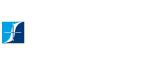HOME SEARCH BYMOTOR TYPE SEARCH BYINDUSTRY PROCESS & RESULTS SERVICES MOTOR RESOURCES CONTACT

 Motor Resources

# Online Calculators

Given torque in any unit, and speed in RPM, calculate power in watts [W] and horsepower [hp].

Conversion Calculators
The following online calculators will convert to/from any of the units listed below:

Torque Units [dyn·cm] dyne centimeter, [µN·m] micronewton meter, [mN·m] millinewton meter, [N·cm] newton centimeter, [N·m] Newton meter, [kN·m] kilonewton meter, [MN·m] meganewton meter, [g·cm] gram-force centimeter, [kg·cm] kilogram-force centimeter, [kg·m] kilogram-force meter, [oz·in] ounce-force inch, [oz·ft] ounce-force foot, [lb·in] pound-force inch, [lb·ft] pound-force foot

[W] watt, [kW] kilowatt, [hp] horsepower (550 ft·lbf / s), horsepower (electric), horsepower (metric), [CV] cheval vapeur (France), [PS] pferdestarke (Germany), horsepower (U.K.)

Force Units [dyn] dyne, [N] Newton, [kN] kilonewton, [gf] gram-force, [kgf] kilogram-force, [tf] ton-force (metric), [ozf] ounce-force, [lbf] pound-force, kip (1000 lbf), ton-force (short; 2000 lbf), ton-force (long; 2240 lbf)

Mass Units [g] gram, [kg] kilogram, [t] tonne / metric ton (in US), [oz] ounce, [LB] pound, [tn] short ton (2000 LB), long ton
(2240 LB)

Length Units [mm] millimeter, [cm] centimeter, [m] meter, [in] inch, [ft] feet

[K] Kelvin, [°C] degrees Celsius, [°F] degrees Fahrenheit

Inertia Units [kg·cm²] kilogram centimeter squared, [kg·m²] kilogram meter squared, [oz·in²] ounce inch squared, [lb·in²] pound inch squared, [lb·ft²] pound foot squared, [oz·in·s²] ounce inch per second squared, [lb·in·s²] pound inch per second squared, [lb·ft·s²] pound foot per second squared